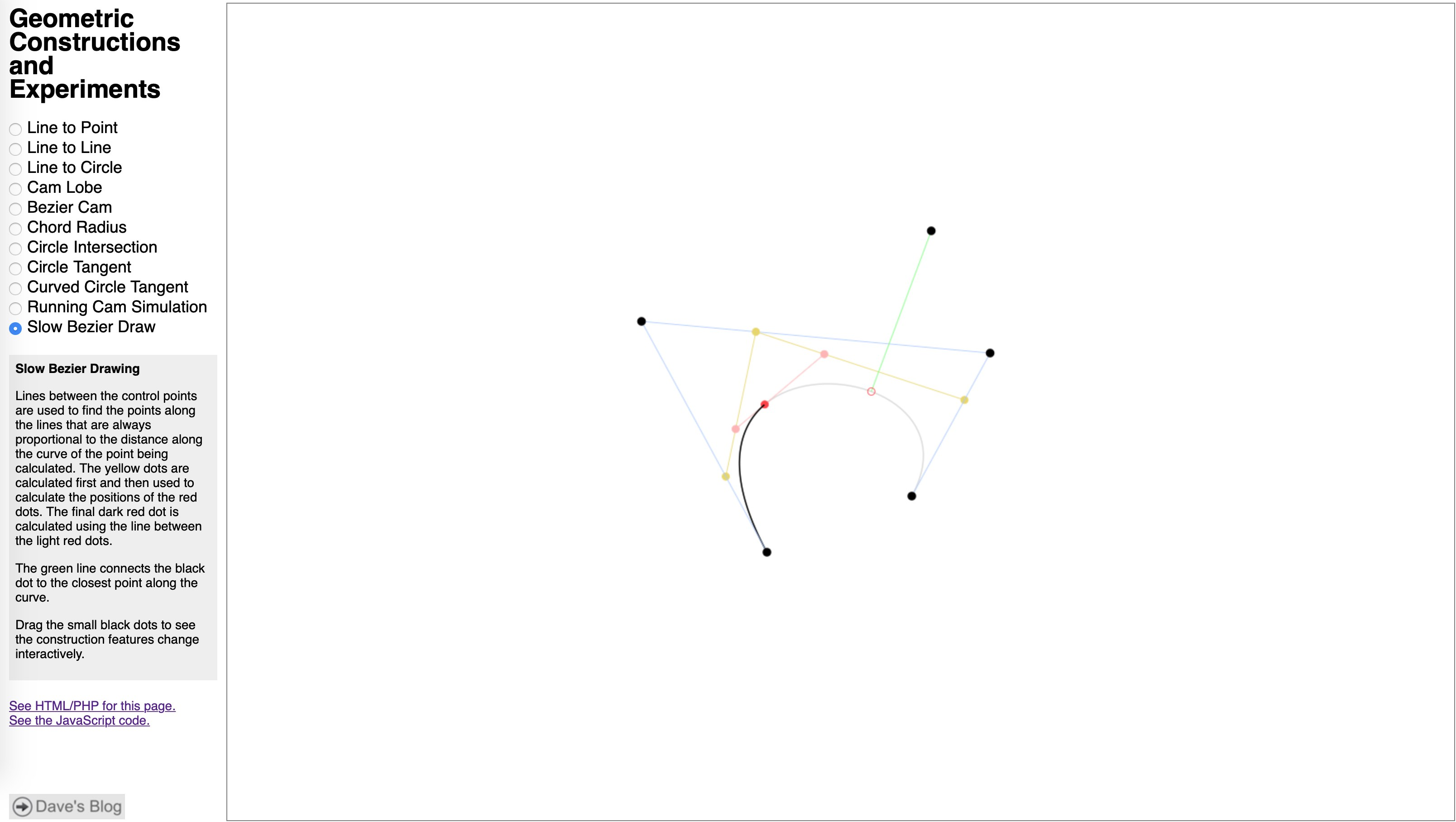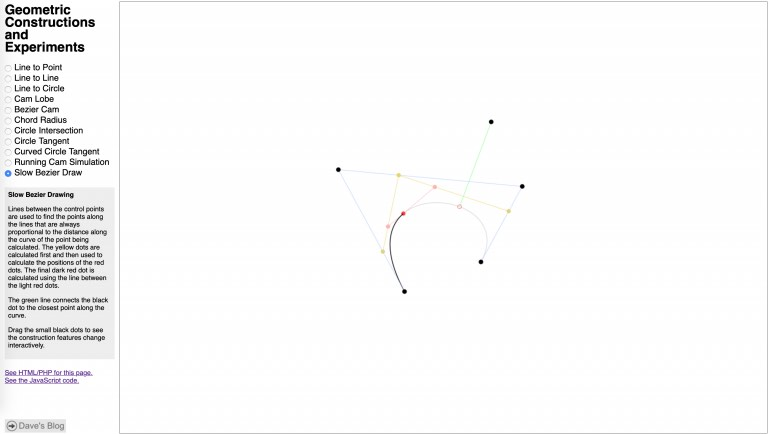I am about to add curve drawing to the Linkage program. The curves will be compound curves, or whatever you would call a chain of elements that can be lines, arcs, or Bezier curves. To start work on this, the first thing I did was remind myself how to draw a cubic Bezier curve that has four control points.

The geometric constructions page shown above is where I’ve done a few different experiments written in JavaScript. All of these use a framework I built for the page that makes some of the work fairly simple.

A cubic Bezier curve uses four control points. Although they are all used mathematically in the same manner, the first and last points end up also being the start and end of the curve and the other two points are never reached by the curve. Watching the animation on the page shown above makes it clear what is happening but I’ll describe it anyhow: To find any point on the curve defined by a number between 0.0 and 1.0 that represents its distance along the curve (1.0 being 100% along the curve), lines are created, mathematically or just in one’s imagination, between the first and second, second and third, and third and fourth points. Call these points A, B, and C. My code creates actual line objects so they can be drawn. Then a point is calculated along each line using that same distance number between 0.0 and 1.0. Lines are then created (in my code for drawing and to keep track of the end points easily) from points A to B and B to C. One last line is created and the point along it is calculated. That last point is the point on the curve as specified by the number between 0.0 and 1.0. To draw the curve, the code runs in a loop and steps through number from 0.0 to 1.0 to draw line segments between those points. The more steps there are, the smaller the segments and the smoother the curve. Optimized drawing code would figure out the number of steps based on the expected length of the segments to get the best looking curve without drawing extra segments.

It’s a lot to describe in text but it’s fairly easy to implement. In fact, al of the code I’ve written so far has been fairly simple.

To compute the point along the curve, it is better to use a little math and not actually create lines, real or imaginary. The code above draws the Bezier curve on my test page. I got the point calculation from somewhere on the internet – it is fairly common and seems to be the most optimal way to get the point along the curve. If you try downloading the code from my test page, you will see that I also create the lines and points so I can draw all of them for the construction animation.

The method of finding the closest point on the curve to some other point is purely iterative without any interesting math at all. There is supposed to be something more optimal available somewhere but I dread having to implement code to deal with Sturm’s theorem. My method simply divides the curve a small set of points and checks to see which point is closest to the test point. The code then takes the closest point and divides a section of curve around that point (half way to the point before and after it) into points. Those are all tested and the same thing happens again. I do this a few times to get fairly accurate results. When I implement this in C++ in the Linkage program, I’ll do some performance testing using a profiler and increase or decrease the level of accuracy of the algorithm as need to keep the program running smoothly.

If you are paying attention and have some idea of how the code is working right now, you may notice that the points along the curve that are tested might be far enough apart to cause errors in some situations. The easiest situation to reproduce an error is when the curve crosses itself. In that case, there is always a point that will be fond closest to the wrong point not eh curve.